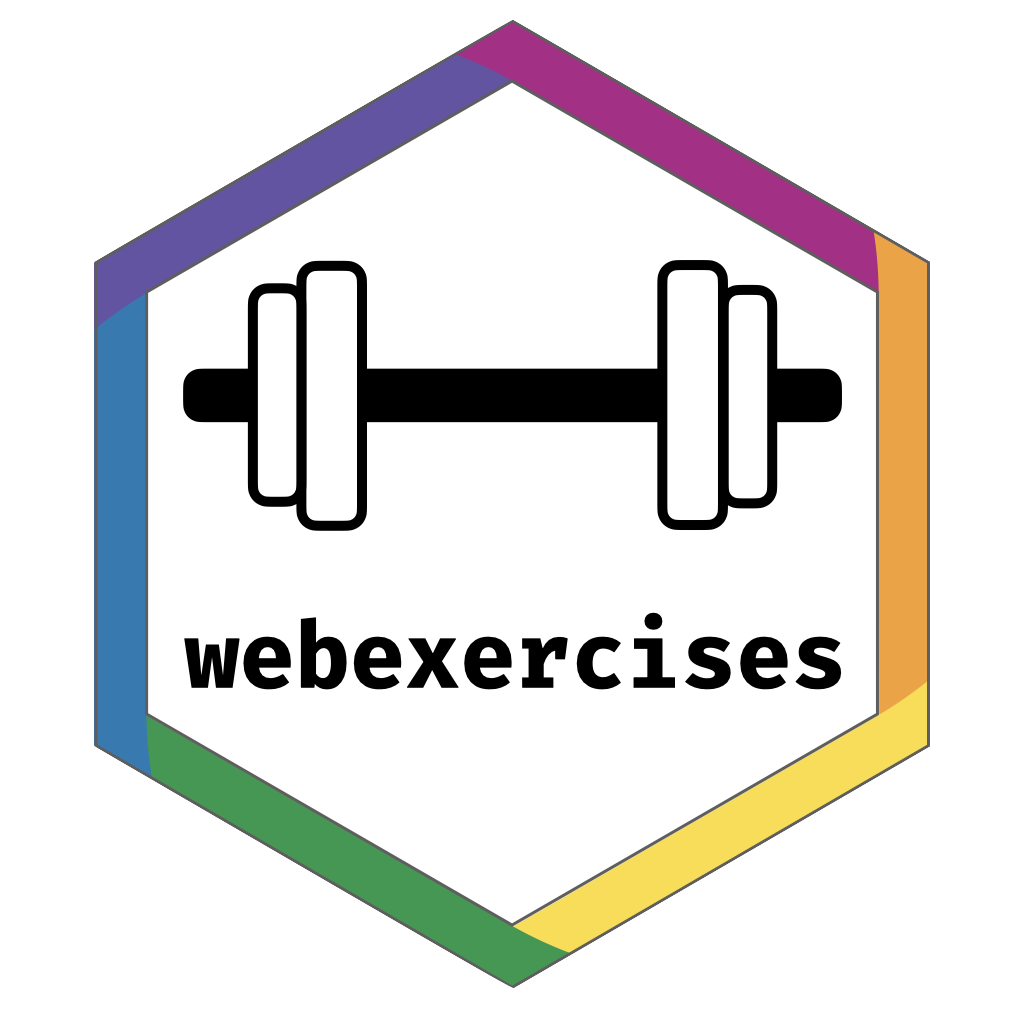The goal of webexercises is to enable instructors to easily create interactive web pages that students can use in self-guided learning. Although webexercises has fewer features than RStudio’s learnr package, it is more lightweight: whereas learnr tutorials must be either hosted on a shiny server or run locally, webexercises creates standalone HTML files that require only a JavaScript-enabled browser. It is also extremely simple to use.

## Installation

You can install webexercises from CRAN using:

install.packages("webexercises")

You can install the development version from GitHub with:

devtools::install_github("psyteachr/webexercises")

## Creating interactive widgets with inline code

The webexercises package provides functions that create HTML widgets using inline R code. These functions are:

function widget description
fitb() text box fill-in-the-blank question
mcq() pull-down menu multiple choice question
torf() pull-down menu TRUE or FALSE question
longmcq() radio buttons MCQs with long answers
hide() and unhide() button solution revealed when clicked
total_correct() text updating total correct

The appearance of the text box and pull-down menu widgets changes when users enter the correct answer. Answers can be either static or dynamic (i.e., specified using R code). Widget styles can be changed using style_widgets().

Examples are provided in the Web Exercises R Markdown template. To create a file from the webexercises template in RStudio, click File -> New File... -> RMarkdown and in the dialog box that appears, select From Template and choose Web Exercises.

Alternatively (or if you’re not using RStudio) use:

rmarkdown::draft("exercises.Rmd", "webexercises", "webexercises")

Knit the file to HTML to see how it works. Note: The widgets only function in a JavaScript-enabled browser.

These functions are optimised to be used with inline r code, but you can also use them in code chunks by setting the chunk option results = 'asis' and using cat() to display the result of the widget.

# echo = FALSE, results = 'asis'
opts <- c("install.package",
"install.packages",
"libraries")

q1 <- mcq(opts)

cat("What function loads a package that is already on your computer?", q1)

### Total Correct

The function total_correct() displays a running total of correct responses. Change the elem argument to display in a different style (e.g., h2 or h3 for header styles). If you’re comfortable with css styles or classes, you can add them with the args argument.

total_correct(elem = "h3", args = "style='color:#003366;'")

### Fill-In-The-Blanks

Create fill-in-the-blank questions using fitb(), providing the answer as the first argument.

fitb(4)
• 2 + 2 is

You can also create these questions dynamically, using variables from your R session (e.g., in a hidden code chunk).

x <- sample(2:8, 1)
fitb(x)
• The square root of 9 is:

The blanks are case-sensitive; if you don’t care about case, use the argument ignore_case = TRUE.

fitb("E", ignore_case = TRUE)
• What is the letter after D?

If you want to ignore differences in whitespace use, use the argument ignore_ws = TRUE (which is the default) and include spaces in your answer anywhere they could be acceptable.

fitb(c("library( tidyverse )", "library( \"tidyverse\" )", "library( 'tidyverse' )"), ignore_ws = TRUE, width = "20")
• How do you load the tidyverse package?

You can set more than one possible correct answer by setting the answers as a vector.

fitb(c("A", "E", "I", "O" , "U"), ignore_case = TRUE)
• Type a vowel:

You can use regular expressions to test answers against more complex rules.

fitb("^[a-zA-Z]{3}\$", width = 3, regex = TRUE)
• Type any 3 letters:

### Multiple Choice

Set up a multiple-choice drop-down menu using mcq().

mcq(c("tidyr", "dplyr", answer = "readr", "ggplot2"))
• What package helps you load CSV files?
• “Never gonna give you up, never gonna:
• “I down in Africa” -Toto

### True or False

Make quick true/false questions with torf().

torf(TRUE)
torf(FALSE)
• True or False? You can permute values in a vector using sample().

### Longer MCQs

When your answers are very long, sometimes a drop-down select box gets formatted oddly. You can use longmcq() to deal with this. Since the answers are long, It’s probably best to set up the options inside an R chunk with echo=FALSE.

opts_p <- c(
"the probability that the null hypothesis is true",
answer = "the probability of the observed, or more extreme, data, under the assumption that the null-hypothesis is true",
"the probability of making an error in your conclusion"
)
longmcq(opts_p)

What is a p-value?

What is true about a 95% confidence interval of the mean?

### Hidden solutions and hints

You can fence off a solution area that will be hidden behind a button using hide() before the solution and unhide() after, each as inline R code. Pass the text you want to appear on the button to the hide() function.

hide("Click here to see the solution")
unhide()

If the solution is an RMarkdown code chunk, instead of using hide() and unhide(), you can set the webex.hide chunk option to TRUE, or set it to the string you wish to display on the button.

library(tidyverse)

## Bookdown

You can add webexercises to a bookdown project or start a new bookdown project using add_to_bookdown().

# create a new book
# use default includes and scripts directories (include and R)
output_format = "bs4_book",
render = TRUE)

output_format = "gitbook",
render = TRUE)

output_format = "html_book",
render = TRUE)

output_format = "tufte_html_book",
render = TRUE)

# update an existing book with custom include and script directories
include_dir = "www",
script_dir = "scripts",
output_format = "gitbook")

## Learnr syntax

You can use learnr syntax to set up webexercises, too.

quiz(caption = "Learnr Syntax Examples",
# fitb: a single true answer
question("2 + 2 is",
question("Type a vowel:",
type = "learnr_text",
options = list(ignore_case = TRUE)),
# mcq: short answers (charlength <= 50)
question("Never gonna give you up, never gonna:",
)